1800-1023-196

+91-120-4616500

# Molar Conductivity or Molar Conductance

## Molar Conductivity or Molar Conductance

Molar conductivity is defined as the conducting power of all the ions produced by dissolving one mole of an electrolyte in solution.

It is denoted by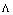(lambda). Molar conductance is related to specific conductance (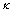) as,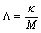where, M is the molar concentration.

If M is in the units of molarity i.e., moles per litre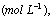themay be expressed as,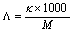For the solution containing 1 gm mole of electrolyte placed between two parallel electrodes of 1 sq. cm area of cross-section and one cm apart,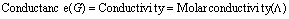But if solution contains 1 gm mole of the electrolyte therefore, the measured conductance will be the molar conductivity. Thus,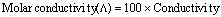In other words,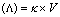where V is the volume of the solution in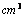containing one gram mole of the electrolyte.

If M is the concentration of the solution in mole per litre, then

M mole of electrolyte is present in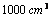1 mole of electrolyte is present in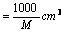of solution

Thus,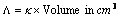containing 1 mole of electrolyte.

or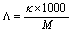Units of Molar Conductance: The units of molar conductance can be derived from the formula ,The units ofare S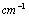and units ofare,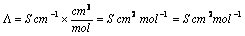According to SI system, molar conductance is expressed as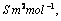if concentration is expressed as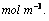## NEET & AIIMS Exam Sample Papers

 AIIMS SAMPLE PAPERS View More NEET SAMPLE PAPERS View More